# Scope Creep - Damned if I Do, Damned if I Don't

Dec. 14, 2022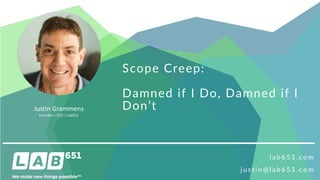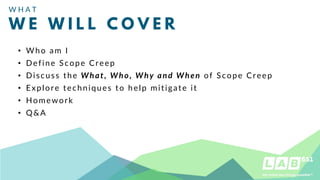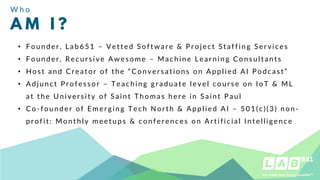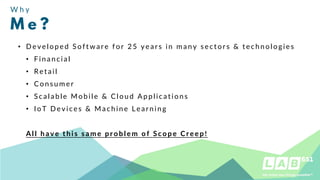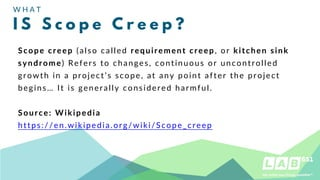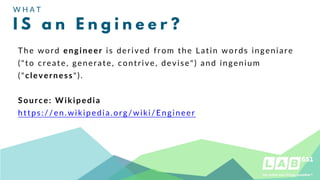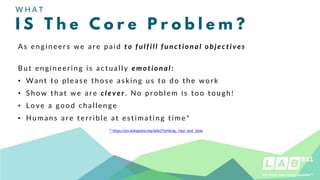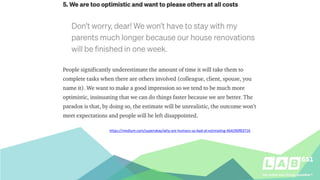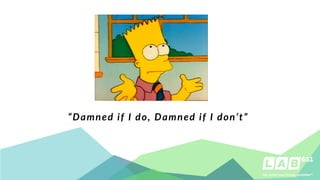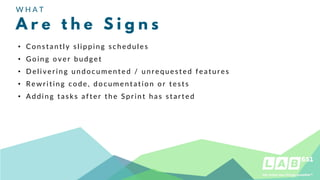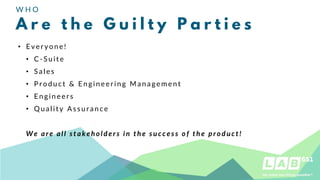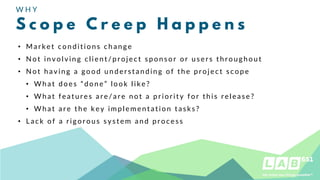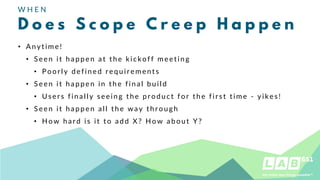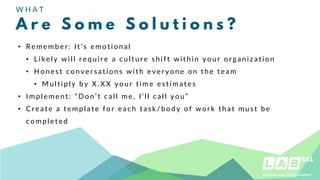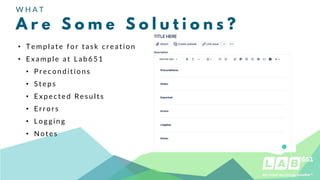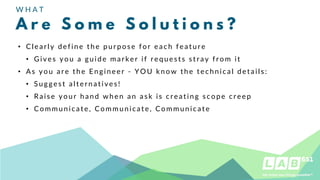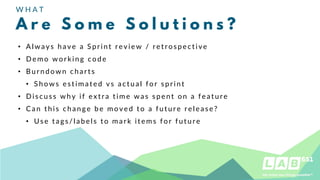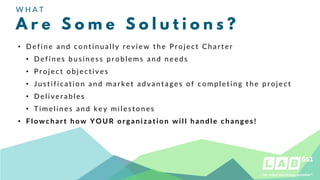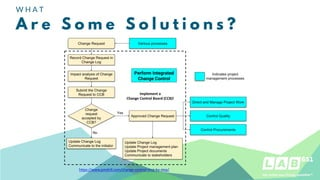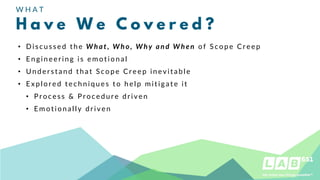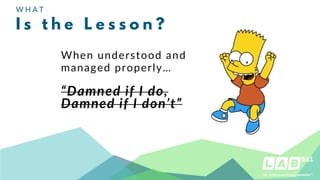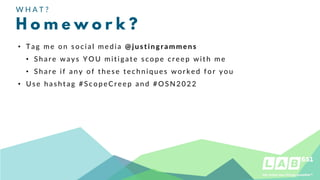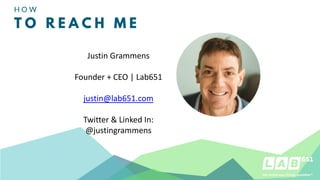1 of 23

### Scope Creep - Damned if I Do, Damned if I Don't

1. Scope Creep: Damned if I Do, Damned if I Don’t l a b 6 5 1 . c o m j u s t i n @ l a b 6 5 1 . c o m Justin Grammens Founder + CEO | Lab651
2. • Who am I • Define Scope Creep • Discuss the What, Who, Why and When of Scope Creep • Explore techniques to help mitigate it • Homework • Q&A W H A T W E W I L L C O V E R
3. W h o A M I ? • F ou n der, Lab651 – Ve t t e d S o f t war e & P r o je ct S t af f in g S e r v ice s • F ou n der, Recu rs ive Awes om e – M ach in e Le arn in g Co n s u lt an t s • Ho s t an d C reat or of t h e “ C on v ers at ion s on Applied AI P odcas t ” • Adju n ct P r o f e s s o r – Te ach in g g radu at e le v e l co u rs e o n I o T & M L at t h e Un iv ers it y of S ain t Th om as h ere in S ain t P au l • C o-f ou n der of Em ergin g Tech Nort h & Applied AI – 501(c)(3) n on- prof it : M on t h ly m eet u ps & con f eren ces on Art if icial I n t elligen ce
4. W h y M e ? • D e veloped Sof t ware f or 25 ye ars in m an y s e ct or s & t ech n ologies • F in an cial • Ret ail • C on s u m er • S calable M obile & C lou d Applicat ion s • I oT D evices & M ach in e Learn in g A l l have thi s s ame probl e m of Scope Cre e p!
5. W H A T I S S c o p e C r e e p ? Scope creep (also called requirement creep, or kitchen sink syndrome) Refers to changes, continuous or uncontrolled growth in a project’s scope, at any point after the project begins… It is generally considered harmful. Source: Wikipedia https://en.wikipedia.org/wiki/Scope_creep
6. W H A T I S a n E n g i n e e r ? The word engineer is derived from the Latin words ingeniare ("to create, generate, contrive, devise") and ingenium ("cleverness"). Source: Wikipedia https://en.wikipedia.org/wiki/Engineer
7. W H A T I S T h e C o r e P r o b l e m ? As e n g in e e r s we ar e paid t o f ulf i ll f unct i onal ob je ct i v e s B u t en gin eerin g is act u ally e m ot i onal: • Wan t t o pleas e t h os e as k in g u s t o do t h e work • S h ow t h at we are cle v e r. No problem is t oo t ou gh ! • Lo ve a good ch allen ge • Hu m an s are t e rrible at e s t im at in g t im e* * https://en.wikipedia.org/wiki/Thinking,_Fast_and_Slow
9. “Damned if I do, Damned if I don’t”
10. W H A T A r e t h e S i g n s • C on s t an t ly s lippin g s ch edu les • Goin g over bu dget • D e live rin g u n docu m en t ed / u n re qu e s t e d f e at u re s • Rewrit in g code, docu m en t at ion or t es t s • Addin g t as k s af t e r t h e S pr in t h as s t ar t e d
11. W H O A r e t h e G u i l t y P a r t i e s • E veryon e! • C -S u it e • S ales • P rodu ct & En gin eerin g M an agem en t • E n gin eers • Q u alit y As s u ran ce W e are all s t ake ho lde rs i n t he s ucce s s o f t he p ro duct !
12. W H Y S c o p e C r e e p H a p p e n s • M arke t con dit ion s ch an g e • No t in volvin g clien t / pro je ct s pon s or or u s ers t h ro u g h ou t • No t h avin g a good u n ders t an din g of t h e project s cope • Wh at does “ don e” look lik e? • Wh at f eat u res are/ are n ot a priorit y f or t h is releas e ? • Wh at are t h e k ey im plem en t at ion t as k s ? • Lack o f a rigorou s s ys t e m an d pr oces s
13. W H E N D o e s S c o p e C r e e p H a p p e n • An y t im e ! • S een it h appen at t h e k ick of f m eet in g • P oorly def in ed requ irem en t s • S een it h appen in t h e f in al bu ild • Us ers f in ally s eein g t h e produ ct f or t h e f irs t t im e - yikes ! • S een it h appen all t h e way t h rou gh • Ho w h ard is it t o add X? How abou t Y ?
14. W H A T A r e S o m e S o l u t i o n s ? • Rem em ber: I t ’ s em ot ion al • Likely w ill requ ire a cu lt u re s h if t wit h in you r organ iz at ion • Ho n es t con v ers at ion s wit h e veryon e on t h e t eam • M u lt iply by X.XX you r t im e e s t im at e s • I m plem en t : “ D on ’ t call m e, I ’ ll call you ” • C reat e a t em plat e f or each t as k / body of work t h at m u s t be com plet ed
15. W H A T A r e S o m e S o l u t i o n s ? • Te m plat e f o r t as k cre at io n • E xam ple at Lab651 • P recon dit ion s • S t eps • E xpect ed Res u lt s • E rrors • Loggin g • No t es
16. W H A T A r e S o m e S o l u t i o n s ? • C learly def in e t h e pu rpos e f or each f eat u re • Gives you a gu ide m arker if requ es t s s t ray f rom it • As y o u ar e t h e E n g in e e r - Y O U k n ow t h e t ech n ical det ails : • S u gges t alt ern at iv es ! • Rais e y ou r h an d wh en an as k is creat in g s cope creep • C om m u n icat e, C om m u n icat e, C om m u n icat e
17. W H A T A r e S o m e S o l u t i o n s ? • Alway s h av e a S pr in t r e v ie w / r e t r o s pe ct iv e • D e m o workin g code • B u rn down ch art s • S h ows es t im at ed v s act u al f or s prin t • D is cu s s wh y if ext ra t im e was s pe n t on a f e at u re • C an t h is ch an ge be m ov ed t o a f u t u re releas e? • Us e t ags / labels t o m ark it em s f or f u t u re
18. W H A T A r e S o m e S o l u t i o n s ? • D e f in e an d con t in u ally revie w t h e P ro je ct C h art er • D e f in es bu s in es s problem s an d n e e ds • P roject object ives • Ju s t if icat io n an d m ark e t adv an t ag e s o f co m ple t in g t h e pro je ct • D e liverables • Tim e lin e s an d k e y m ile s t o n e s • F l owchart how Y O UR organiz ation wil l handl e change s !
19. W H A T A r e S o m e S o l u t i o n s ? https://www.pmdrill.com/change-control-step-by-step/ Implement a Change Control Board (CCB)!
20. W H A T H a v e W e C o v e r e d ? • D is cu s s ed t h e W hat , W ho , W hy and W he n of S cope C reep • E n gin eerin g is em ot ion al • Un ders t an d t h at S cope C reep in evit able • E xplored t ech n iqu es t o h elp m it igat e it • P roces s & P rocedu re driven • E m ot ion ally driven
21. W H A T I s t h e L e s s o n ? When understood and managed properly… “Damned if I do, Damned if I don’t”
22. W H A T ? H o m e w o r k ? • Tag m e o n s o cial m e dia @ j us ti ngra mme ns • S h are way s Y O U m it igat e s cope creep wit h m e • S h are if an y of t h es e t ech n iqu es work ed f or y ou • Us e h as h t ag #S copeC reep an d #O S N2022
23. H O W T O R E A C H M E Justin Grammens Founder + CEO | Lab651 justin@lab651.com Twitter & Linked In: @justingrammens